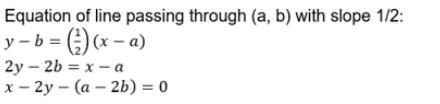Courses

# Test: Various Forms Of Equation Of A Line

## 10 Questions MCQ Test Mathematics For JEE | Test: Various Forms Of Equation Of A Line

Description
This mock test of Test: Various Forms Of Equation Of A Line for JEE helps you for every JEE entrance exam. This contains 10 Multiple Choice Questions for JEE Test: Various Forms Of Equation Of A Line (mcq) to study with solutions a complete question bank. The solved questions answers in this Test: Various Forms Of Equation Of A Line quiz give you a good mix of easy questions and tough questions. JEE students definitely take this Test: Various Forms Of Equation Of A Line exercise for a better result in the exam. You can find other Test: Various Forms Of Equation Of A Line extra questions, long questions & short questions for JEE on EduRev as well by searching above.
QUESTION: 1

### Which of the following lines is parallel to the line with equation 2x+y=3?

Solution:

This question can be done by picking the options.
a1/a2 = b1/b2 = c1/c2
Equation : 2x + y = 3
(d) 4x + 2y = 6
a1 = 2⇒, b1 = 1, c1 = 3
a2 = 4, b2 = 2, c2 = 6
⇒ 2/4 = 1/2 = 3/6
=> 1/2 = 1/2 = 1/2

QUESTION: 2

### The equation of the line having normal distance 2a from the origin and angle 60° which the normal makes with the positive direction of X-axis is:

Solution:

We know that, If p is the length of the normal from origin to a line and w is the angle made by the normal with the positive direction of x-axis then the equation of the line is given by xcosw + ysinw = p.
Here, p=2a units and w=60°
Thus, the required equation of the given line is
xcos30° + ysin30° = 2a
x(1/2) + y(√3/2) = 2a
x + √3y = 4a

QUESTION: 3

### The equation of a line with x intercept 4 and y intercept 3 is given by

Solution:
QUESTION: 4

Find the equation of the line whose intercepts on X and Y-axes are a2 and brespectively

Solution:

Consider the given points. (a2,0) and (0,b2)
We know that the equation of the line which is passing through the points
y−y1 =[ (y2−y1) / (x2−x1)] (x−x1)
So, y−0 = b2−0/0-a2(x-a2)
-ya2 - b2x = -b2a2
xb2 +ya2 = b2a2

QUESTION: 5

The equations of the line parallel to X-axis and at a distance of c/2 from the X-axis is:

Solution:

The equation of straight line is parallel to x-axis which means slope is 0
equation of straight line , y = mx + c
here, m = 0 , y = c
As it is given c = c/2
y = +-c/2

QUESTION: 6

The line which makes intercepts 3 and 4 on the x and the y axis respectively, has equation

Solution: Intercept form is
x/a +y/b =1
in this question
x/3 +y/4=1
by solving this equation, we get
4x + 3y =12
QUESTION: 7

Find the equation of line passing through the mid-point of line joining the points (3, 4) and (5, 6) and perpendicular to the equation of line 2x + 3y = 5.

Solution:

Mid Point [(3+5)/2, (4+6)/2]
= [8/2, 10/2]
= (4,5)
Perpendicular to the equation : 2x + 3y - 5 = 10
3x - 2y + k = 0
3(4) - 2(5) + k = 0
12 - 10 + k  0
k = -2
Therefore the equation is : 3x - 2y - 2 = 0

QUESTION: 8

A line is known with its slope and an intercept on one of the axes, then the equation of the line is in the form of:

Solution:

The slope-intercept form of a linear equation is written as y = mx + b, where m is the slope and b is the value of y at the y-intercept, which can be written as (0, b).

QUESTION: 9

For the line x+y = 1, what is the angle made with the positive direction of the x axis?

Solution:

We have in Equation = mx+c the slope of line as m and m=tanθ, θ is the angle made by (+ve)  in X-axis
Hence,  y = 1 - x
Here m = -1 = tanθ
θ = 135o

QUESTION: 10

The equation of the line through (a, b) with slope 1/2 is:

Solution: